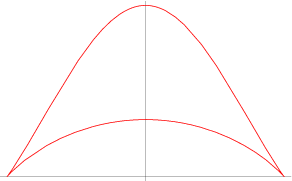JavaScript 数学曲线—双角线

简介绘制function draw() {

let a = -100, start = 0;

let x = 0, y = 0, points = [];

const acceleration = 0.1, max = 40;

while (start <= max) {

x = a * Math.sin(start);

const yNumerator = a * Math.pow(Math.cos(start), 2) * (2 + Math.cos(start));

const yDenominator = 3 + Math.pow(Math.sin(start), 2);

y = yNumerator / yDenominator;

points.push([x, y]);

start = start + acceleration;

}

// 实现把点绘制成线的方法

line({ points: points});

}

参考资料